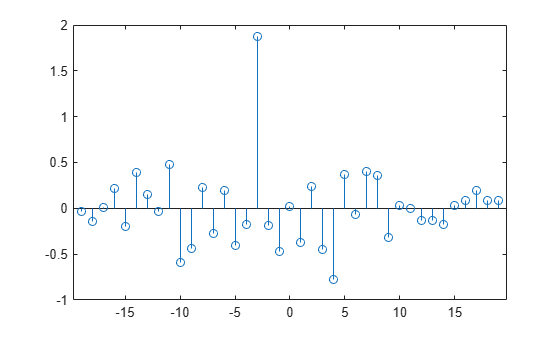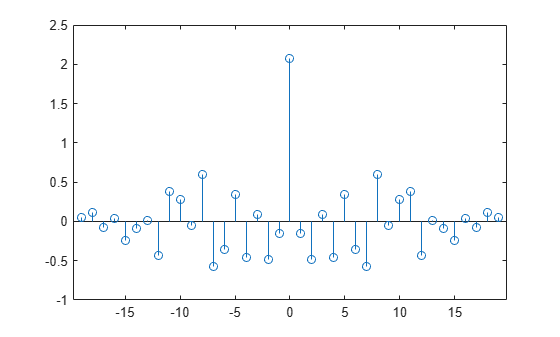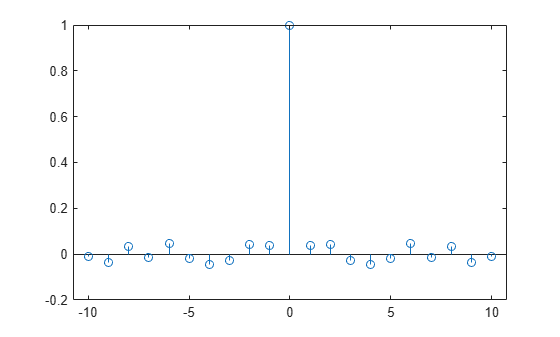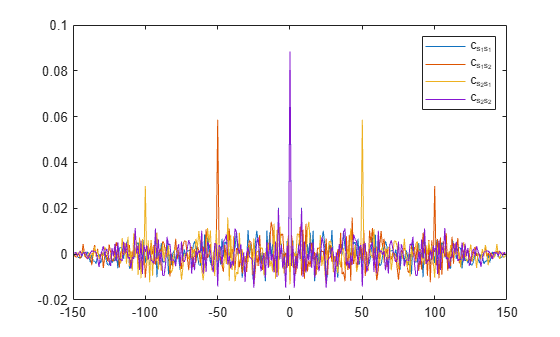xcov

Cross-covariance

Description

example

c = xcov(x,y) returns the cross-covariance of two discrete-time sequences. Cross-covariance measures the similarity between a vector x and shifted (lagged) copies of a vector y as a function of the lag. If x and y have different lengths, the function appends zeros to the end of the shorter vector so it has the same length as the other.

example

c = xcov(x) returns the autocovariance sequence of x. If x is a matrix, then c is a matrix whose columns contain the autocovariance and cross-covariance sequences for all combinations of the columns of x.

example

c = xcov(___,maxlag) sets the lag range from -maxlag to maxlag for either of the previous syntaxes.

example

c = xcov(___,scaleopt) also specifies a normalization option for the cross-covariance or autocovariance. Any option other than 'none' (the default) requires the inputs x and y to have the same length.

example

[c,lags] = xcov(___) also returns the lags at which the covariances are computed.

Examples

collapse all

Create a vector of random numbers x and a vector y that is equal to x shifted by 3 elements to the right. Compute and plot the estimated cross-covariance of x and y. The largest spike occurs at the lag value when the elements of x and y match exactly (-3).

rng default
x = rand(20,1);
y = circshift(x,3);
[c,lags] = xcov(x,y);
stem(lags,c)Create a 20-by-1 random vector, then compute and plot the estimated autocovariance. The largest spike occurs at zero lag, where the vector is exactly equal to itself.

rng default
x = rand(20,1);
[c,lags] = xcov(x);
stem(lags,c)Compute and plot the estimated autocovariance of white Gaussian noise, $c\left(m\right)$, for $-10\le m\le 10$. Normalize the sequence so that it is unity at zero lag.

rng default
x = randn(1000,1);
maxlag = 10;
[c,lags] = xcov(x,maxlag,'normalized');
stem(lags,c)Create a signal made up of two signals that are circularly shifted from each other by 50 samples.

rng default
shft = 50;
s1 = rand(150,1);
s2 = circshift(s1,[shft 0]);
x = [s1 s2];

Compute and plot biased estimates of the autocovariance and mutual cross-covariance sequences. The output matrix c is organized as four column vectors such that $c=\left(\begin{array}{cccc}{c}_{{s}_{1}{s}_{1}}& {c}_{{s}_{1}{s}_{2}}& {c}_{{s}_{2}{s}_{1}}& {c}_{{s}_{2}{s}_{2}}\end{array}\right)$. ${\mathit{c}}_{{\mathit{s}}_{1}{\mathit{s}}_{2}}$ has maxima at -50 and +100 and ${\mathit{c}}_{{\mathit{s}}_{2}{\mathit{s}}_{1}}$ has maxima at +50 and -100 as a result of the circular shift.

[c,lags] = xcov(x,'biased');
plot(lags,c)
legend('c_{s_1s_1}','c_{s_1s_2}','c_{s_2s_1}','c_{s_2s_2}')Input Arguments

collapse all

Input array, specified as a vector, matrix, or multidimensional array. If x is a multidimensional array, then xcov operates column-wise across all dimensions and returns each autocovariance and cross-covariance as the columns of a matrix.

Data Types: single | double
Complex Number Support: Yes

Input array, specified as a vector.

Data Types: single | double
Complex Number Support: Yes

Maximum lag, specified as an integer scalar. If you specify maxlag, the returned cross-covariance sequence ranges from -maxlag to maxlag. By default, the lag range equals 2N – 1, where N is the greater of the lengths of inputs x and y.

Data Types: single | double

Normalization option, specified as one of the following.

• 'none' — Raw, unscaled cross-covariance. 'none' is the only valid option when inputs x and y have different lengths.

• 'biased' — Biased estimate of the cross-covariance.

• 'unbiased' — Unbiased estimate of the cross-covariance.

• 'normalized' or 'coeff' — Normalizes the sequence so that the autocovariances at zero lag equal 1.

Output Arguments

collapse all

Cross-covariance or autocovariance, returned as a vector or matrix.

If x is an M × N matrix, then xcov(x) returns a (2M – 1) × N2 matrix with the autocovariances and cross-covariances of the columns of x. If you specify a maximum lag maxlag, then the output c has size (2 × maxlag + 1) × N2.

For example, if S has three columns, $\text{S}=\left(\begin{array}{ccc}{x}_{1}& {x}_{2}& {x}_{3}\end{array}\right)$, then the result of C = xcov(S) is organized as

$\text{c}=\left(\begin{array}{lllllllll}{c}_{{x}_{1}{x}_{1}}\hfill & {c}_{{x}_{1}{x}_{2}}\hfill & {c}_{{x}_{1}{x}_{3}}\hfill & {c}_{{x}_{2}{x}_{1}}\hfill & {c}_{{x}_{2}{x}_{2}}\hfill & {c}_{{x}_{2}{x}_{3}}\hfill & {c}_{{x}_{3}{x}_{1}}\hfill & {c}_{{x}_{3}{x}_{2}}\hfill & {c}_{{x}_{3}{x}_{3}}\hfill \end{array}\right).$

Lag indices, returned as a vector.

collapse all

Cross-Covariance and Autocovariance

xcov computes the mean of its inputs, subtracts the mean, and then calls xcorr.

The result of xcov can be interpreted as an estimate of the covariance between two random sequences or as the deterministic covariance between two deterministic signals.

The true cross-covariance sequence of two jointly stationary random processes, xn and yn, is the cross-correlation of mean-removed sequences,

${\varphi }_{xy}\left(m\right)=E\left\{\left({x}_{n+m}-{\mu }_{x}\right){\left({y}_{n}-{\mu }_{y}\right)}^{\ast }\right)\right\},$

where μx and μy are the mean values of the two stationary random processes, the asterisk denotes complex conjugation, and E is the expected value operator. xcov can only estimate the sequence because, in practice, only a finite segment of one realization of the infinite-length random process is available.

By default, xcov computes raw covariances with no normalization:

${c}_{xy}\left(m\right)=\left\{\begin{array}{ll}\sum _{n=0}^{N-m-1}\left({x}_{n+m}-\frac{1}{N}\sum _{i=0}^{N-1}{x}_{i}\right)\left({y}_{n}^{\ast }-\frac{1}{N}\sum _{i=0}^{N-1}{y}_{i}^{\ast }\right),\hfill & m\ge 0,\hfill \\ {c}_{yx}^{\ast }\left(-m\right),\hfill & m<0.\hfill \end{array}$

The output vector c has elements given by

$\text{c(m)}={c}_{xy}\left(m-N\right),\text{ }m=1,\dots ,2N-1.$

The covariance function requires normalization to estimate the function properly. You can control the normalization of the correlation by using the input argument scaleopt.

 Orfanidis, Sophocles J. Optimum Signal Processing: An Introduction. 2nd Edition. New York: McGraw-Hill, 1996.

 Larsen, Jan. “Correlation Functions and Power Spectra.” November, 2009. https://www2.imm.dtu.dk/pubdb/edoc/imm4932.pdf